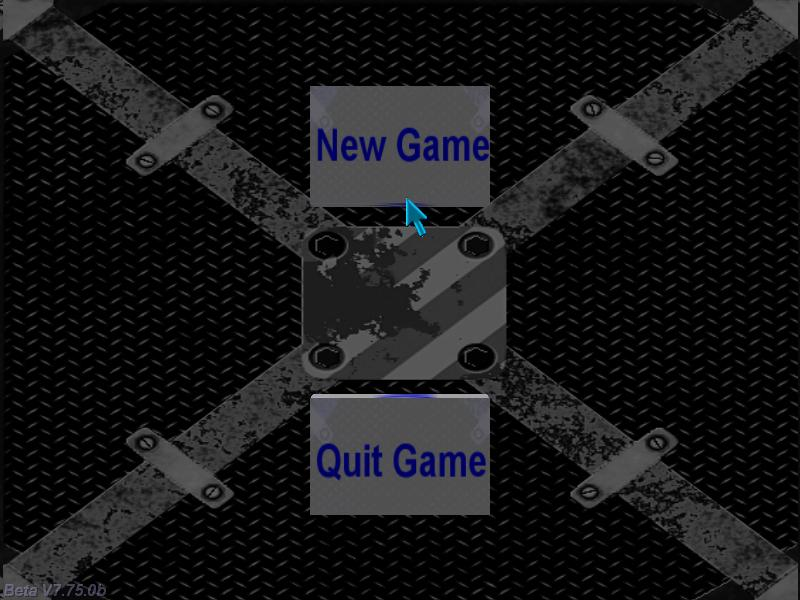Questions from the forum
 Q: Is it possible to create a high precision timer for my racing game? A: Use the snippet below:   FONT* arial_font = "Arial#20";   var hours; var minutes; var seconds; var milliseconds;   function timer_startup() {        while (1)        {                milliseconds += (timer() / 1000);                if (milliseconds > 1000)                {                        milliseconds -= 1000;                        seconds += 1;                }                if(seconds > 59)             {                     minutes += 1;                        seconds -= 60;                        if(minutes >= 59)                        {                                hours += 1;                           minutes -= 60;                        }                }                wait (1);        } }   PANEL* timer_pan = {        layer = 15;        digits(100, 20, 2, arial_font , 1, hours);        digits(150, 20, 2, arial_font , 1, minutes);        digits(200, 20, 2, arial_font , 1, seconds);        digits(250, 20, 3, arial_font , 1, milliseconds);        flags = SHOW; }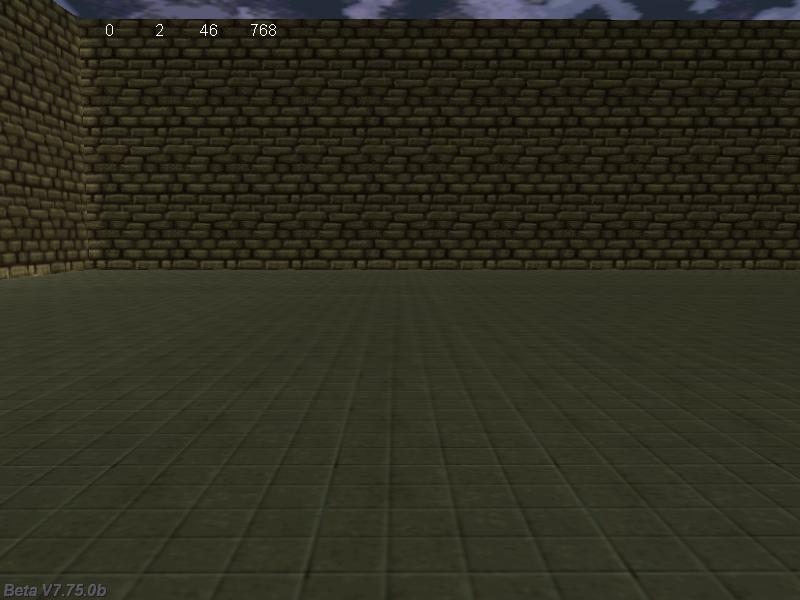Q: Does anyone know a way to make an entity appear at a spot on the level where a user clicks? A: Use this example:   BMAP* pointer_tga = "pointer.tga";   STRING* target_mdl = "dot.mdl";   function adjust_target() {        my.z += 5; // play with the target entity here         }   function create_target() {        VECTOR pos1, pos2;        pos1.x = mouse_pos.x;        pos1.y = mouse_pos.y;        pos1.z = 0;        vec_for_screen (pos1, camera);        pos2.x = mouse_pos.x;        pos2.y = mouse_pos.y;        pos2.z = 20000; // use a big value here        vec_for_screen (pos2, camera);        c_trace (pos1.x, pos2.x, IGNORE_PASSABLE); // now "target" holds the coordinates of the hit point        ent_create (target_mdl, target, adjust_target); // create the entity at the "target" position }   function mouse_startup() {         on_mouse_left = create_target; // create a target entity when the player presses the left mouse button        mouse_mode = 2;        mouse_map = pointer_tga;        while (1)        {                 vec_set(mouse_pos, mouse_cursor);                wait(1);        } }     Q: Is there any other way to detect entity collision other than using event_type? I'd like to track a collision only with a particular door. A: You can use event_type to check if an entity has collided only with a particular door, just like this:   ENTITY* my_door;   action special_door() // attach this action to your door {        my_door = my; }   function detect_door() {        if (you == my_door)        {                beep(); // beep twice only if the player has collided with the particular door                beep();        } }   action players_code() // attach this action to your player {        var movement_speed = 10; // movement speed        VECTOR temp;        set (my, INVISIBLE); // 1st person player        player = my; // I'm the player        my.emask |= (ENABLE_IMPACT | ENABLE_ENTITY);        my.event = detect_door;        while (1)        {                my.pan -= 7 * mouse_force.x * time_step;                camera.x = my.x;                camera.y = my.y;                camera.z = my.z + 50 + 1.1 * sin(my.skill44); // play with 50 and 1.1                camera.pan = my.pan;                camera.tilt += 5 * mouse_force.y * time_step;                vec_set (temp.x, my.x); // trace 10,000 quants below the player                temp.z -= 10000;                temp.z = -c_trace (my.x, temp.x, IGNORE_ME | IGNORE_PASSABLE | USE_BOX) - 2; // play with 2                temp.x = movement_speed * (key_w - key_s) * time_step;                temp.y = movement_speed * (key_a - key_d) * 0.6 * time_step;                c_move (my, temp.x, nullvector, IGNORE_PASSABLE | GLIDE);                wait (1);        } }     Q: How can I make an entity move to the specified location? A: Here's an example that moves an NPC character towards its goal using primitive, and yet fully functional obstacle avoidance code.   ENTITY* goal;   action npc_goal() // attach this action to the destination entity {        goal = my;         }   action goal_tracker() // attach this action to your NPC character {        while (!goal) {wait (1);} // wait until the goal entity is loaded        VECTOR temp, temp_angle;        var npc_speed = 5;        var covered_dist, i;        while (1)        {                my.skill10 += 6 * time_step; // 6 gives the animation speed                vec_set (temp.x, my.x); // trace 10,000 quants below the npc entity                temp.z -= 10000;                temp.z = -c_trace (my.x, temp.x, IGNORE_ME | IGNORE_PASSABLE | USE_BOX) + 20; // play with 20                temp.x = npc_speed * time_step;                temp.y = 0;                ent_animate(my, "walk", my.skill10, ANM_CYCLE);                covered_dist = c_move (my, temp.x, nullvector, IGNORE_PASSABLE | GLIDE);                if (covered_dist < 0.1) // the npc is stuck?                {                        my.pan += 90 - random(180); // then add a random angle to its pan angle                        i = 0;                        while (i < 10) // walk in the new direction for 10 frames, play with 10                        {                                c_move (my, temp.x, nullvector, IGNORE_PASSABLE | GLIDE);                                ent_animate(my, "walk", my.skill10, ANM_CYCLE);                                i++;                                wait (1);                        }                                                }                else // the npc can move? Then rotate it towards the goal again!                {                        vec_set(temp_angle, goal.x);                        vec_sub(temp_angle, my.x);                        vec_to_angle(my.pan, temp_angle);                        my.tilt = 0;                }                if (vec_dist(goal.x, my.x) < 50) // the npc has found the goal entity?                        break; // then get out of the while loop!                wait (1);        }        // the goal was reached here        ent_animate(my, "stand", 0, 0); // switch to "stand" }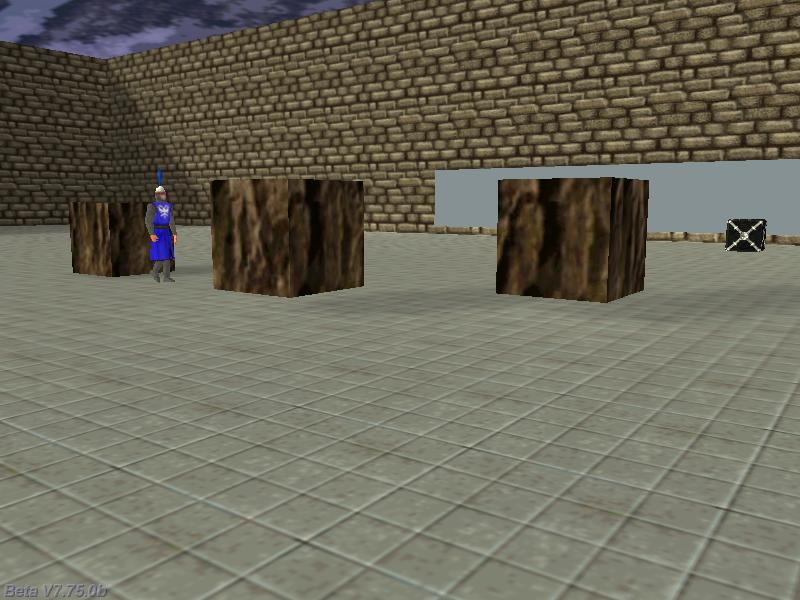Q: I have a car model; how do I make it follow the direction of the path smoothly? A: Here's a simplified version of the code used in my car demo.   #define car_speed skill55   SOUND* aiskids1_wav = "aiskids1.wav"; SOUND* carai1_wav = "carai1.wav";   ENTITY* dummy1; ENTITY* carai1_dummy; ENTITY* carai1;   action car_ai() {        carai1 = my;        var distance = 0;        var previous_pan;        var following_pan;        var min_speed = 20;        var max_speed;        var carai_engine;        var engine_factor;        var dist_z;        var skids_once = 0;        VECTOR last_pos;        VECTOR direction;        VECTOR temp;        max_speed = 35;        dummy1 = ent_create(NULL, nullvector, NULL);        // create another (carai1_dummy) dummy entity that will move on the path        // the ai car will use the same x, y, pan, tilt and roll with the carai1_dummy entity that moves on the path        // but will compute its own z using c_trace; this way, the nodes don't have to be place precisely above the ground        carai1_dummy = ent_create(NULL, nullvector, NULL);        path_set(dummy1, "path_001"); // make sure to name your path this way        engine_factor = 2 + random(2); // start with a random engine sound frecquency each and every time        carai_engine = ent_playloop(carai1, carai1_wav, 300); // start playing the car ai engine sound in a loop        while(1)        {                previous_pan = carai1.pan;                // place the carai1 entity on the path                path_spline(dummy1, carai1_dummy.x, distance);                distance += dummy1.car_speed * time_step;                // let carai1 look ahead                vec_diff(direction, carai1_dummy.x, last_pos);                vec_to_angle(carai1_dummy.pan, direction);                vec_set(last_pos, carai1_dummy.x);                carai1.pan = carai1_dummy.pan;                carai1.tilt = carai1_dummy.tilt;                carai1.roll = carai1_dummy.roll;                carai1.x = carai1_dummy.x;                carai1.y = carai1_dummy.y;                vec_set (temp.x, carai1_dummy.x);                temp.z -= 2000;                dist_z = c_trace(carai1_dummy.x, temp.x, IGNORE_ME | IGNORE_PASSABLE | IGNORE_MODELS); // ignore the "real" carai                carai1.z = carai1_dummy.z - dist_z + 35; // 35 = experimental value                // sets a variable car ai engine frequency, depending on the speed of the car                snd_tune(carai_engine, 0, dummy1.car_speed * engine_factor, 0);                wait(1);                following_pan = carai1.pan;                if (abs(following_pan - previous_pan) > 1) // sudden direction change? Then lower the speed of the AI car                {                        dummy1.car_speed -= 4 * time_step;                        if (skids_once == 0)                                                {                                skids_once = 1;                                if (random(1) > 0.9)                                {                                        ent_playsound(dummy1, aiskids1_wav, 3000);                                }                        }                }                else                {                        skids_once = 0;                        dummy1.car_speed += 2 * time_step;                }                dummy1.car_speed = clamp(dummy1.car_speed, min_speed, max_speed);        } }     Q: How can I make a bitmap stay full screen, regardless of the screen resolution? A: Use this snippet:   BMAP* splash_tga = "splash.tga"; // splash screen bitmap   PANEL* splash_pan = {        bmap = splash_tga;        layer = 15;        flags = SHOW; }   // makes the panel fill the entire screen, regardless of the screen size and / or the bitmap resolution function panel_startup() {        while (1)        {                splash_pan.scale_x = screen_size.x / bmap_width(splash_tga);                splash_pan.scale_y = screen_size.y / bmap_height(splash_tga);                wait (1);        } }     Q: I wonder if it is possible to make an installer of the game I created. How can I do that? A: There are several free installers that get the job done; here's how Inno Setup from http://www.jrsoftware.org/ works: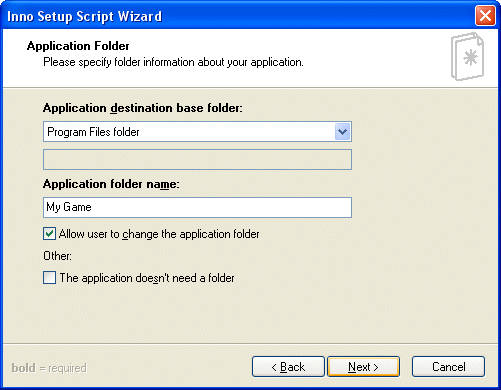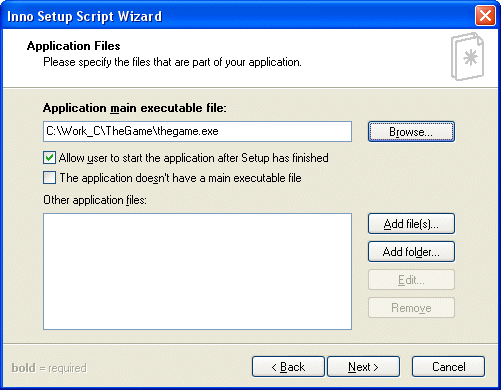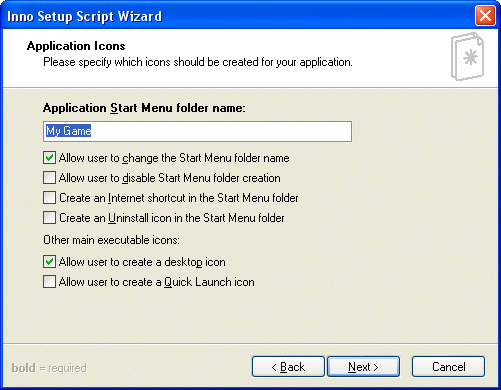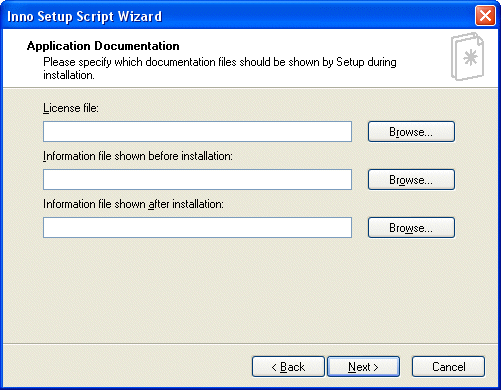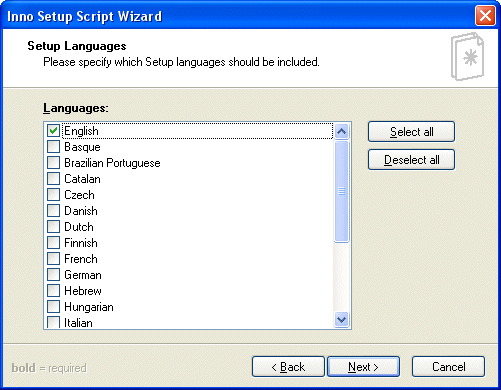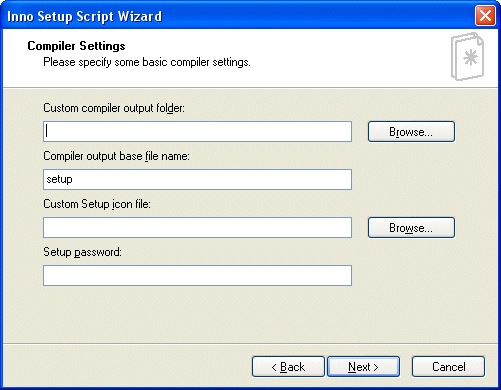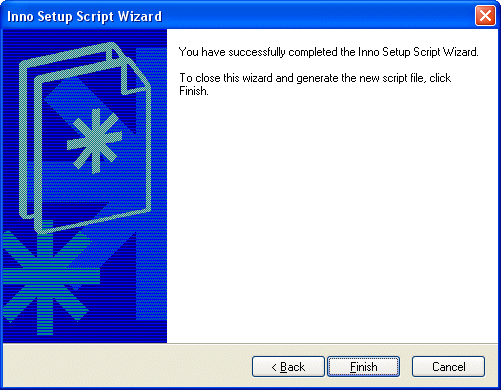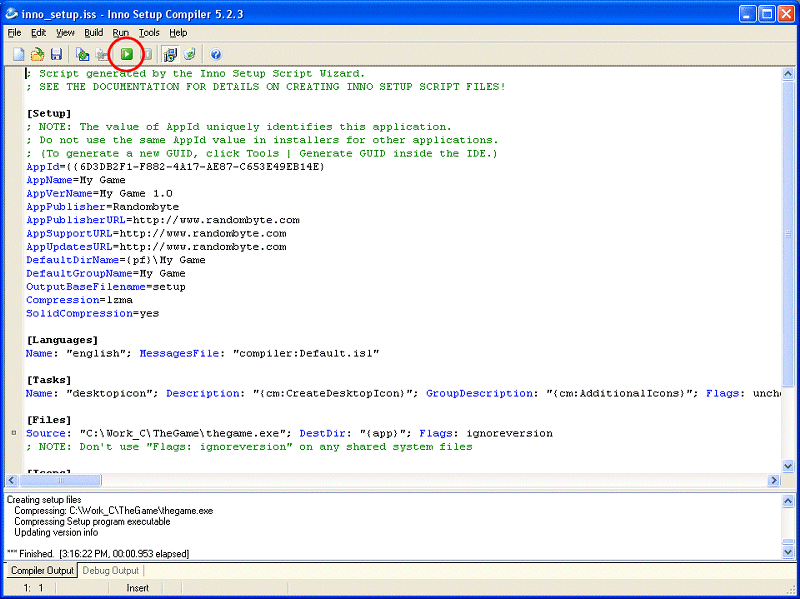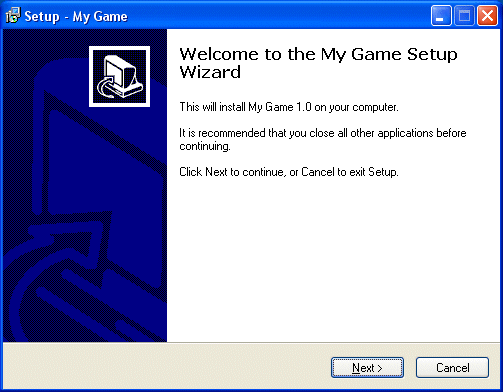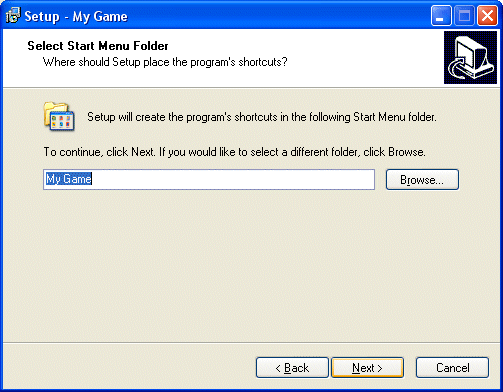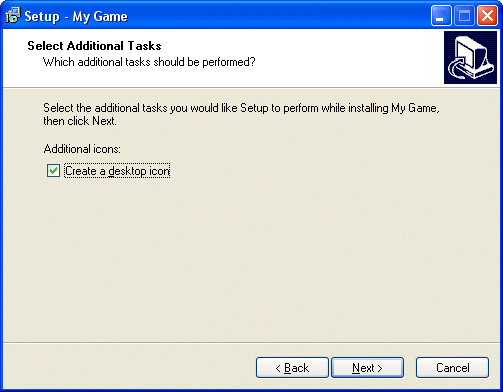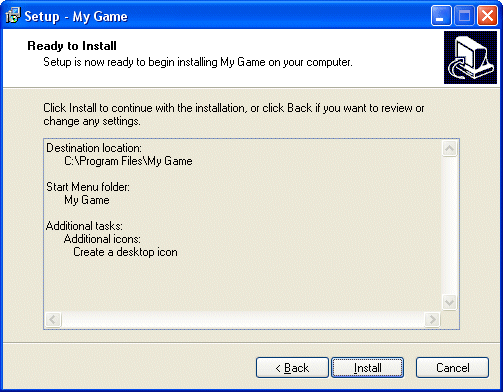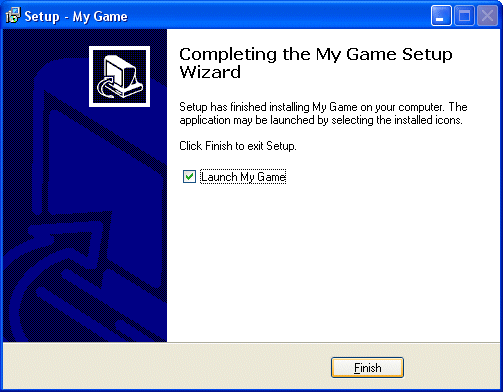Q: I want to have a light moving on a path. How can I do that? A: Use this snippet:   var entity_speed = 3; var movement_enabled = 0; var dist_to_node; var current_node = 1;   VECTOR temp_angle; VECTOR pos_node; // stores the position of the node   function move_target() {        while(1)        {                if(movement_enabled)                {                        entity_speed = minv(5, entity_speed + 0.5 * time_step);                        c_move(my, vector(entity_speed * time_step, 0, 0), nullvector, IGNORE_PASSABLE | GLIDE);                        vec_to_angle (my.pan, vec_diff (temp_angle, pos_node, my.x));                }                wait(1);        } }   action light_follows_path() // attach this action to your light model {  //        set (my, INVISIBLE | PASSABLE); // if you don't want the light to be visible        vec_set(my.blue, vector(255, 255, 255)); // set a white color for the light        my.lightrange = 300; // and a range of 300 quants        move_target();        result = path_scan(me, my.x, my.pan, vector(360, 0, 500)); // scan the area        if (result) {movement_enabled = 1;}        path_getnode (my, 1, pos_node, NULL);        vec_to_angle (my.pan, vec_diff (temp_angle, pos_node, my.x)); // rotate towards the node        while(1)        {                dist_to_node = vec_dist(my.x, pos_node);                if(dist_to_node < 50) // close to the node?                {                        current_node = path_nextnode(my, current_node, 1);                        if (!current_node) {current_node = 1;} // reached the end of the path? Then start over!                        path_getnode (my, current_node, pos_node, NULL);                }                wait(1);        } }     Q: I'm looking for a jump-pad snippet like in Quake. A: There you go:   SOUND* jump_wav = "jump.wav";   function jump_now() {        snd_play(jump_wav, 80, 0);        my.event = NULL; // don't trigger several jumps at once        var vertical_force = 100; // play with 100        while(vertical_force > 1) //while we have some jump force left        {                if (player) // we are controlling the player?                {                        c_move(player, vector(0, 0, vertical_force * time_step), nullvector, 0);                }                vertical_force -= 2.5 * time_step; // play with 2.5                wait(1);        }        my.event = jump_now; // allow the jumps again }   action jumping_pad() // attach this action to your jump pad model / wmb entity {        my.emask |= (ENABLE_IMPACT | ENABLE_ENTITY);        my.event = jump_now; }   action players_code() // need special player code as well {        var movement_speed = 10; // movement speed        VECTOR temp;        set (my, INVISIBLE); // 1st person player        player = my; // I'm the player        while (1)        {                my.pan -= 7 * mouse_force.x * time_step;                camera.x = my.x;                camera.y = my.y;                camera.z = my.z + 50 + 1.1 * sin(my.skill44); // play with 50 and 1.1                camera.pan = my.pan;                camera.tilt += 5 * mouse_force.y * time_step;                temp.x = movement_speed * (key_w - key_s) * time_step;                temp.y = movement_speed * (key_a - key_d) * 0.6 * time_step;                temp.z = -15 * time_step;                c_move (my, temp.x, nullvector, IGNORE_PASSABLE | GLIDE);                wait (1);        } }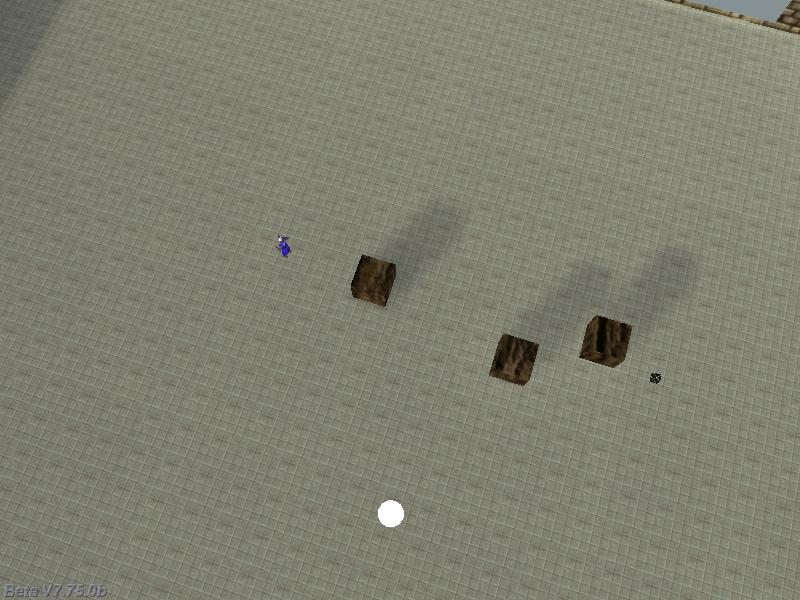Q: How can I create a 3D menu, with 3D buttons that can be clicked? A: Use this example as a base for your code:   BMAP* pointer_tga = "pointer.tga";   function mouse_startup() {         mouse_mode = 2;        mouse_map = pointer_tga;        while (1)        {                 vec_set(mouse_pos, mouse_cursor);                wait(1);        } }   function start_game() {        printf("Starts a new game"); }   function new_game() {        my.emask |= ENABLE_CLICK;        my.event = start_game; }   function end_game() {        sys_exit (NULL); // shut down the engine }   function quit_game() {        my.emask |= ENABLE_CLICK;        my.event = end_game; }   function main() {        vec_set(screen_color, vector(200, 0, 0)); // make the background color blue        fps_max = 70;        video_mode = 7; // run in 800x600 pixels        video_depth = 32; // 32 bit mode        level_load (NULL); // load an empty level        wait (2); // wait a bit        camera.pan = 90; // point the camera in the right direction        ent_create("background.mdl", vector (0, 200, 0), NULL); // create the background model        ent_create("newgame.mdl", vector (0, 135, 30), new_game); // create the "New game" model        ent_create("quitgame.mdl", vector (0, 135, -30), quit_game); // create the "Quit game" model }